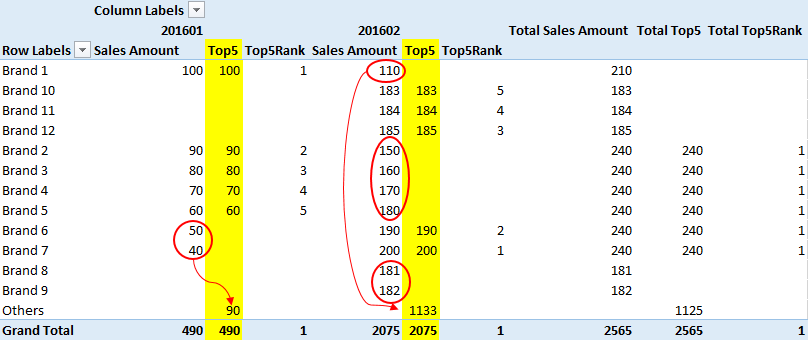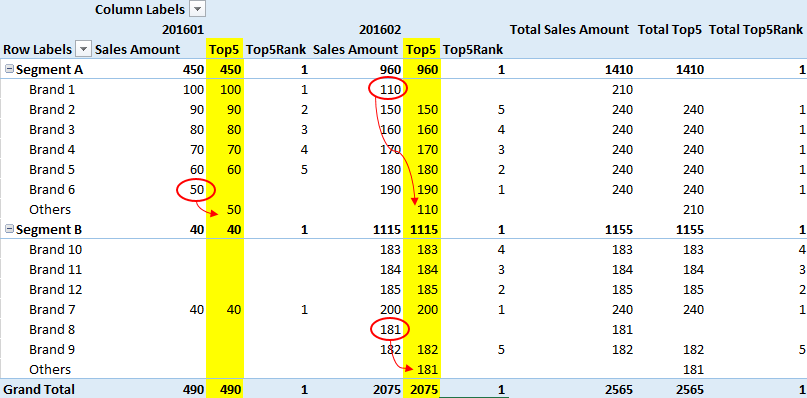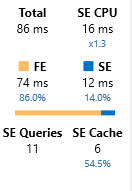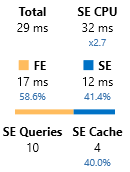Saturday, June 4, 2016 #

## DAX - Creating a measure for Top 5 and Others

Often when you have a requirement to show a measure for the TopN of a given attribute the users will want to see the subtotal of all the other items.

With multi-dimensional cubes and MDX you can create calculated members in a dimension. But you can’t do this in a tabular model using DAX . You could create a calculated column, but then your TopN would be fixed and could not respond dynamically to changes in filters as calculated columns are calculated and stored at processing time.

So one way to make this work is to have a placeholder row (or rows) with "Others" in the table which you are doing the TopN over.

The first step in this technique is to create a Rank measure (this saves having to re-type the rank logic multiple times). In this example I’m doing a top 5 Brands.

Brand Rank:=RANKX ( ALL ( Brand[BrandName] ), [Sales Amount] )

Then we create a top 5 measure that returns the sales amount if the rank is less than or equal to 5 and will return the sum of all ranks if the current brand is "Others" otherwise it will return BLANK()

Top5 :=
IF (
[Brand Rank] <= 5,
[Sales Amount],
IF (
HASONEVALUE ( Brand[BrandName] ),
IF (
VALUES ( Brand[BrandName] ) = "Others",
SUMX ( FILTER ( ALL ( Brand[BrandName] ), [Brand Rank] > 5 ), [Sales Amount] )
)
)
)

Then if we create a pivot table with this measure we get the following result. In the picture below I’m showing the raw Sales amount so you can see how the amounts get re-assigned in the Top5 calculation.This also works as you change the pivot table. When we introduce the Segment on the rows we can show a figure for “Others” within each segment. (Note that for this to work I had to create 2 placeholder “Others” rows, one in each segment). There is a link at the bottom of this post to the file that these screenshots were taken from.At this point, I’m pretty happy. It seems to be working well although calculating ranks is rather expensive so I’m wondering how it will work against larger data sets. I know that there are definitely ways to make this faster if you are using the current generation of DAX engines (Excel 2016, Power BI or SQL 2016) as we have access to things like variables and the INTERSECTS function which we could use in conjunction with TOPN. But I think there are also opportunities to make this faster with “v1” DAX

Running this algorithm against the Adventure Works Product Name column results in a performance profile like the following (using the server timings feature of DAX Studio)Which shows 86% of the time being spent in the formula engine. I’m pretty sure that a fair bit of this time is spent calculating ranks greater than 5 for the “Others” calculation. So I thought it might be faster to a TOPN in descending order and get everything except for the first 5. The formula for that is the following

Top5v2 :=
IF (
[Brand Rank] <= 5,
[Sales Amount],
IF (
HASONEVALUE ( Brand[BrandName] ),
IF (
VALUES ( Brand[BrandName] ) = "Others",
SUMX (
TOPN (
COUNTROWS ( ALL ( Brand[BrandName] ) ) - 5,
ALL ( Brand[BrandName] ),
[Sales Amount], 1
),
[Sales Amount]
)
)
)

The modified version of the calculation has a much better performance profile, taking about 1/3 of the time and with an almost 50/50 split between the Formula Engine and the Storage Engine.